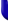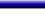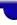Lighthouse3d.com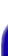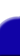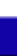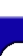Terrain Tutorial Index A TGA Library A Simple TGA Library TGA lib Source Height Maps Height Maps from Images Artificial Terrain Generation The Fault Algorithm Implementation Details Two Simple Variations The Circles Algorithm Smoothing Smoothing Matrix filters API details# Terrain Tutorial

## Particle Deposition

I first came across this algorithm in the book "Graphic Programming Gems" (an excellent book btw). The algorithms presented in here are a merge between the book's idea and something I saw on the web at "Pulchritudinous Primes". Anyway, here goes.

The main idea behind particle deposition is ... particle deposition. What that means is that we're going to start with a flat grid and add "particles", one by one, until we're pleased with the general shape of the terrain. By adding a particle, I actually mean adding a vertical displacement at a grid point.

To start with, a random grid point (x,z) is selected. The height of the selected grid point, i.e. its y component, is increased by a certain amount. The displacement can be constant, or a decreasing value as we iterate.After the grid point is displaced, we need to select another point. Use up your imagination to select the next point, there are so many alternatives. The approach taken in here is a very simple one, and its based on the site mentioned above: a random number between 1 and 4 is generated, and the value represents a direction. An example is shown bellow:

```    ```
v = rand();
switch(v) {
case 1: x++;break; // move right
case 2: x--;break; // move left
case 3: z++;break; // move closer
case 4: z--;break; // move away
}
```
```

Then we displace the new point (x,z) upwards. There is just a small detail now. Assume that the particle is placed on a grid point A. Furthermore, assume that one of A's neighbours has a height which is lower than A was before the particle was dropped. Then the particle will roll to the neighbour. The basic idea is to get a "stable" system. With this approach the surface of the terrain will look smooth. The other approach is to have "sticking particles", i.e. a particle stays where it lands regardless of the neighbours heights. The sticking particles approach is more prone to generate peaks. Depending on the effect you're looking for you may choose one of the above approaches.

If we keep adding particles for, say 1000 iterations, we get some interesting shapes as shown bellow: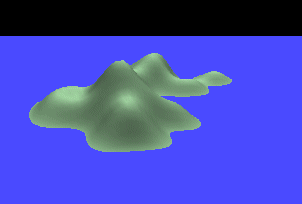and with sticking particles we get,Note: In the terrains above the height was scaled up and a smoothing filter was applied.

As the figures above show the sticking particles method tends to create rougher terrains than the rolling particles. Just one more thing, when the direction found with the method above takes you to a point outside the terrain, I've found that is better to treat the terrain as if it wraps around, rather than moving the point back inside the terrain. However that's just a hint, try it yourself.

As opposed to the previous algorithms, we get interesting effects when we restart. This is because when we start over, we start with an unrelated a fresh random point, and is quite possible that the new point will land far from the "island" shown in the above figure, therefore creating another island. The following terrain was generated with 5 series of 1000 iterations of the algorithm above.As you probably figured out by now there are many possible variations on how to select the next grid point, here are a few although I'm sure that none is original:

a) Select a point and a radius. Generate random points within the circle centered in the first point.

b) Create a black and white image with the same dimension as your terrain. Place black patches in the image in the shape you want your islands to have. Then use that image as a filter to select the next point, i.e. only deposit particles on the terrain if the corresponding image point is black.

c) make up your own and let me know if you get interesting results.

In the source code, namely in the terrain.cpp file, you'll find two functions directly relating to this algorithm. The first sets the particle mode:

void terrainSetParticleMode(int mode);

Parameters:
mode - STICK or ROLL

The second function is the terrain creation function:

int terrainIterateParticleDeposition(int numIt);

Parameters:
numIt - the number of iterations to perform